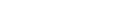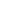xDouble beta decayEncyclopedia
Double beta decay is a radioactive decay process where a nucleus releases two beta rays as a single process.

In double-beta decay, two neutrons in the nucleus are converted to protons, and two electrons and two electron antineutrinos are emitted. In the process of beta minus decay, unstable nuclei
Atomic nucleus
The nucleus is the very dense region consisting of protons and neutrons at the center of an atom. It was discovered in 1911, as a result of Ernest Rutherford's interpretation of the famous 1909 Rutherford experiment performed by Hans Geiger and Ernest Marsden, under the direction of Rutherford. The...

decay by converting a neutron
Neutron
The neutron is a subatomic hadron particle which has the symbol or , no net electric charge and a mass slightly larger than that of a proton. With the exception of hydrogen, nuclei of atoms consist of protons and neutrons, which are therefore collectively referred to as nucleons. The number of...

in the nucleus to a proton
Proton
The proton is a subatomic particle with the symbol or and a positive electric charge of 1 elementary charge. One or more protons are present in the nucleus of each atom, along with neutrons. The number of protons in each atom is its atomic number....

and emitting an electron
Electron
The electron is a subatomic particle with a negative elementary electric charge. It has no known components or substructure; in other words, it is generally thought to be an elementary particle. An electron has a mass that is approximately 1/1836 that of the proton...

and an electron antineutrino. In order for beta decay to be possible, the final nucleus must have a larger binding energy than the original nucleus. For some nuclei, such as germanium-76, the nucleus with atomic number one higher has a smaller binding energy, preventing beta decay from occurring. However, the nucleus with atomic number two higher, selenium-76, has a larger binding energy, so the double-beta decay process is allowed. This process is allowed because the Q-value of the first beta reaction is less than the Q-value of the second beta reaction.

Although efforts to observe the process date back to 1948, it was not observed in a laboratory setting until 1986. Geochemical observation of the decay products (by extraction of Kr
Krypton
Krypton is a chemical element with the symbol Kr and atomic number 36. It is a member of Group 18 and Period 4 elements. A colorless, odorless, tasteless noble gas, krypton occurs in trace amounts in the atmosphere, is isolated by fractionally distilling liquified air, and is often used with other...

and Xe
Xenon
Xenon is a chemical element with the symbol Xe and atomic number 54. The element name is pronounced or . A colorless, heavy, odorless noble gas, xenon occurs in the Earth's atmosphere in trace amounts...

from very old Se
Selenium
Selenium is a chemical element with atomic number 34, chemical symbol Se, and an atomic mass of 78.96. It is a nonmetal, whose properties are intermediate between those of adjacent chalcogen elements sulfur and tellurium...

and Te minerals) are known since 1950. Double-beta decay is the rarest known kind of radioactive decay; it has been observed for only 12 isotopes, and all of them have a mean lifetime of more than 1019 yr.

For some nuclei, the process occurs as conversion of two protons to neutrons, with emission of two electron neutrinos and absorption of two orbital electrons (double electron capture
Double electron capture
Double electron capture is a decay mode of atomic nucleus. For a nuclide with number of nucleons A and atomic number Z, double electron capture is only possible if the mass of the nuclide of is lower....

). If the mass difference between the parent and daughter atoms is more than 1.022 MeV/c2 (two electron masses), another decay branch is accessible, with capture of one orbital electron and emission of one positron
Positron
The positron or antielectron is the antiparticle or the antimatter counterpart of the electron. The positron has an electric charge of +1e, a spin of ½, and has the same mass as an electron...

. When the mass difference is more than 2.044 MeV/c2 (four electron masses), the emission of two positrons is possible. These theoretically possible double-beta decay branches have not yet been observed.

## List of known double-beta decay isotopes

More than 60 naturally occurring isotopes are capable of undergoing double-beta decay. However, only twelve isotopes have been experimentally observed undergoing two-neutrino double-beta decay:

, , ,, , , , , , , , and .

Many isotopes are, in theory, capable both of double-beta decay and other decays. In most cases, the double-beta decay is so rare as to be nearly impossible to observe against the background of other radiation. However, the double-beta decay rate of (also an alpha emitter) has been measured radiochemically; is produced by this type of radioactivity. Two of the nuclides ( and ) from the list above can decay also via single beta decay but this decay is extremely suppressed and has never been observed.

## Neutrinoless double-beta decay

The processes described in the previous section are also known as two-neutrino double-beta decay, as two neutrinos (or antineutrinos) are emitted. If the neutrino is a Majorana particle
Majorana fermion
In physics, a Majorana fermion is a fermion which is its own anti-particle. The term is used in opposition to Dirac fermion, which describes particles that differ from their antiparticles...

(meaning that the antineutrino and the neutrino are actually the same particle), and at least one type of neutrino has non-zero mass (which has been established by the neutrino oscillation
Neutrino oscillation
Neutrino oscillation is a quantum mechanical phenomenon predicted by Bruno Pontecorvowhereby a neutrino created with a specific lepton flavor can later be measured to have a different flavor. The probability of measuring a particular flavor for a neutrino varies periodically as it propagates...

experiments), then it is possible for neutrinoless double-beta decay to occur. In the simplest theoretical treatment of neutrinoless double-beta decay (light neutrino exchange), in essence the two neutrinos annihilate each other, or equivalently, one nucleon
Nucleon
In physics, a nucleon is a collective name for two particles: the neutron and the proton. These are the two constituents of the atomic nucleus. Until the 1960s, the nucleons were thought to be elementary particles...

absorbs the neutrino emitted by another nucleon of the nucleus.

The neutrinos in the above diagram are virtual particles. With only the two electrons in the final state, the total kinetic energy
Kinetic energy
The kinetic energy of an object is the energy which it possesses due to its motion.It is defined as the work needed to accelerate a body of a given mass from rest to its stated velocity. Having gained this energy during its acceleration, the body maintains this kinetic energy unless its speed changes...

of the two electrons would be approximately the difference in binding energy between the initial and final state nuclei (with the recoil of the nucleus accounting for the rest of the available energy). To a very good approximation, the two electrons are emitted back-to-back to conserve momentum
Momentum
In classical mechanics, linear momentum or translational momentum is the product of the mass and velocity of an object...

.

The decay rate for this process is given (approximately) bywhereis the two-body phase-space factor,is the nuclear matrix element, and mββ is the so called effective Majorana neutrino mass given byIn this latter expression, the mi are the neutrino masses (of the ith mass eigenstate), and the Uei are elements of the Pontecorvo–Maki–Nakagawa–Sakata (PMNS) matrix
Pontecorvo–Maki–Nakagawa–Sakata matrix
In particle physics, the Pontecorvo–Maki–Nakagawa–Sakata matrix , Maki–Nakagawa–Sakata matrix , lepton mixing matrix, or neutrino mixing matrix, is a unitary matrixThe PMNS matrix is not unitary in the seesaw model which contains information on the mismatch of quantum states of leptons when they...

. Hence the observation of neutrinoless double-beta decay, in addition to confirming the Majorana nature of the neutrino, would give information on the absolute neutrino mass scale, and potentially also on the neutrino mass hierarchy and the Majorana phases appearing in the PMNS matrix.

### Neutrinoless double-beta decay experiments

Numerous experiments have been carried out to search for neutrinoless double-beta decay. Some recent and proposed future experiments include:
• Completed experiments:

• Current and proposed experiments
• COBRA
• CUORICINO and CUORE
• EXO
Exo
Exo may refer to:*Endo-exo isomerism*The Enriched Xenon Observatory neutrinoless double beta decay experiment*eXo Platform the open source software...

• GERDA
• MAJORANA
• MOON
• NEMO-3
Neutrino Ettore Majorana Observatory
The Neutrino Ettore Majorana Observatory is an international collaboration of scientists searching for neutrinoless double beta decay. The observation of neutrinoless double beta decay would be evidence that neutrinos are Majorana particles and could be used to measure the neutrino mass...

and SuperNEMO
• NEXT
• SNO+
SNO+
SNO+ is an underground physics experiment under construction that makes use of the equipment already installed underground for the former Sudbury Neutrino Observatory experiment at SNOLAB...

• KamLAND-Zen

### Klapdor-Kleingrothaus et al.s claimed observation of neutrinoless double-beta decay

A subgroup of the Heidelberg-Moscow collaboration led by H. V. Klapdor-Kleingrothaus
Hans Volker Klapdor-Kleingrothaus
Hans Volker Klapdor-Kleingrothaus was born on January 25, 1942 in Reinbek and is a German physicist who works in nuclear physics, particle physics and astrophysics.-Biography:...

published a series of controversial papers claiming to have observed neutrinoless double-beta decay in with statistical significance of > 99.9999998%. This claim has been criticized by many in the nuclear and particle physics communities, but Klapdor-Kleingrothaus et al. have vigorously defended their work. Their measured value of mββ between ~0.2-0.5 eV (depending on the choice of nuclear matrix element) has yet to be confirmed by other experiments.

The following lists, in chronological order, the key publications and preprints related to the claim and the criticism thereof.

• Double electron capture
Double electron capture
Double electron capture is a decay mode of atomic nucleus. For a nuclide with number of nucleons A and atomic number Z, double electron capture is only possible if the mass of the nuclide of is lower....

• Beta decay
Beta decay
In nuclear physics, beta decay is a type of radioactive decay in which a beta particle is emitted from an atom. There are two types of beta decay: beta minus and beta plus. In the case of beta decay that produces an electron emission, it is referred to as beta minus , while in the case of a...

• Neutrino
Neutrino
A neutrino is an electrically neutral, weakly interacting elementary subatomic particle with a half-integer spin, chirality and a disputed but small non-zero mass. It is able to pass through ordinary matter almost unaffected...